#### Conics

As a warm up, we start with a standard example y2 = x. When x,y are treated as real variables, this is simply a parabola opening sideways. Things get more interesting when the variables are allowed to take complex values. Since the graph would live in 2 complex or 4 real dimensions, it is impossible visualize completely. Nevertheless, we can get a sense of it projecting to 3 real dimensions, by setting x1 and x2 to be real and imaginary parts of x, and x3 to be the real part of y. ( We will use xi as coordinates of R3.) In polar coordinates, this gives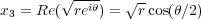We can now plot the graph, using colour to encode the imaginary part of y.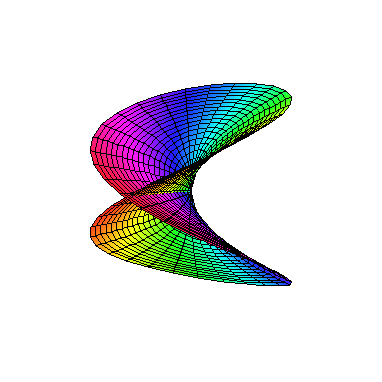Here θ runs from 0 to 4π. Note that even though the surface appears to cross itself. We can see that it doesn't since the colours of sheets are different where they meet.

In general, a conic is given by a quadratic equation f(x,y) = 0. The standard classification that one learns in highschool becomes simpler over the complex numbers, because now there is no difference between an ellipse and a hyperbola. To clarify this, we say that two conics are equivalent if we can transform one to the other by an affine transformation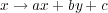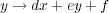where we require the abde matrix to be invertible. Then up to equivalence, there are only 4 possibilities
1. A union of two lines.
2. A double line.
3. The parabola y2 = x.
4. The circle x2 + y2 = 1

We want to give a complex plot of the circle. We start with the usual parameterization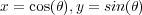and then substitute θ = s + it. From standard identities, we obtain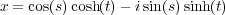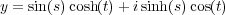Plotting real and imaginary parts of x and the real part of y yields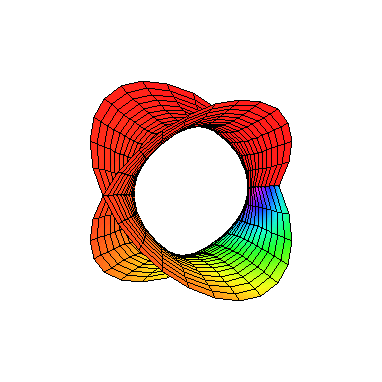The above parameterization of the circle uses transcendental functions. There is another, perhaps less well known, parameterization using only rational functions. Naturally this is referred to as a rational parameterization. The idea is choose a point P on the circle, and parameterize the lines through it by their slope t. These should cut the circle in another point, which can then be expressed in terms of t. Picking P = (0,1), we see that t = (y - 1)∕x. Solving for y and then substituting into the equation for the circle gives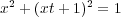Supposing x is nonzero as is typical, we get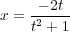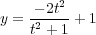We didn’t use these formulas for the plotting, because they are less convenient (we would need t = to complete the circle). However, it has other uses. Suppose that we wanted find all right angle triangles with integer sides. By Pythagoreas’ theorem, this equivalent to finding positive integer solutions for a2 + b2 = c2. Via the substitution x = a∕c, y = b∕c, this is amounts to finding points on the circle with positive rational coordinates. Here’s a very simple procedure: Choose a rational number -1 < t < 0, and substitute into the above formulas.

Return to first page. Go to next page.